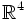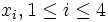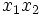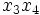Flat torus

Definition

The flat torus in$\R^4$ is defined as the isometric direct product of two circles of equal radius, embedded in orthogonal$\R^2$s.

Equational description

Consider$\R^4$ with coordinates$x_i, 1 \le i \le 4$. The flat torus obtained by taking the direct product of the unit circle is the$x_1x_2$-plane and the unit circle in the$x_3x_4$ plane is defined as the set of points satisfying the following two equations:$x_1^2 + x_2^2 = 1 , \qquad x_3^2 + x_4^2 = 1$

Curvature

The flat torus has zero sectional curvature, on account of being a direct product of two curves. In fact, any surface in$\R^{m+n}$ obtained as an isometric direct product of a curve in$\R^m$ and a curve in$\R^n$, has zero sectional curvature. Template:Justify